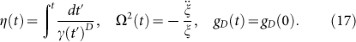## Introduction

“Fast good” is a new culinary concept envisioned by the chef F. Adrià aimed at creating a diet enjoying of two apparently mutually-exclusive features: fast-service and high-quality. When similar ideas are invoked in the quantum realm, one faces the adiabatic theorem which imposes a price. The preparation of a given target state with high-fidelity, free from spurious excitations, generally demands a long time of evolution and the implementation of an adiabatic dynamics. As a result, it comes as no surprise that the recent development of shortcuts to adiabaticity (STA)1, has led to a surge of theoretical2,3,4,5,6,7,8,9,10 and experimental activity11,12,13.

It is the purpose of this work to show that STA can be implemented in a time-dependent box, the paradigmatic model of a quantum piston14. The relevance of this confinement is enhanced by the development of experimental techniques to create optical billiards for ultracold gases15,16, paint arbitrary potential shapes for Bose-Einstein condensates17 and realize all-optical boxes18 and analogous traps in atom chips19. It has the potential to greatly advance the field of quantum simulation, facilitating the connection between ultracold atom experiments and condensed matter systems.

The prospects of finding STA for box confinement seem challenging at the very least, based on the following considerations. Since the early insight by M. Moshinsky in 1952, it is known that the rapid expansion of matter-waves initially localized in a region of space exhibits quantum transients and excitations in the form of density ripples, a phenomenon referred to as diffraction in time20,21,22. Moreover, reflections from the walls of the trap generally lead to Talbot oscillations and the formation of a quantum carpet in the time evolution of the density profile23,24. On top of that, a series of work during the last decades have shown that the suppression of excitations in an expanding box is inevitably constrained by the adiabatic theorem both in the non-interacting25,26,27,28 and mean-field regime29.

In spite of these results preventing ultrafast excitation-free dynamics in box traps, we show that fast non-adiabatic expansion or compression is in fact possible, allowing preparation in a predetermined finite time of the same target state than the adiabatic evolution. The key to this shortcut to adiabaticity is the use of an auxiliary potential term superimposed on the box trap.

We shall start by introducing a dynamical invariant in a time-dependent box trap at the single-particle level and use it to derive a scaling law that governs the time evolution. The inversion of this scaling law will be the key to engineer the STA. This result will be extended to many-body systems with a broad family of interactions. Finally, we shall illustrate the method with some examples relevant to experiments with ultracold gases, showing that the STA is robust in the presence of interactions and experimental imperfections.

## Results

### Dynamical invariants and self-similar dynamics

For a time-dependent box of width ξ(t), the scaling laws governing the dynamics of the expanding eigenstates reported to date are associated with trajectories of the form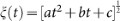(with a, b, c real constants)25,26,27,28, which turn out to be unsuited for engineering a STA (see below). Nonetheless, given a time-dependent Hamiltonian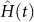, it is possible to build a dynamical invariant30,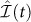such that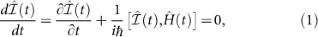with spectral decomposition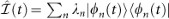in terms of the set of eigenmodes |φn(t)〉 with eigenvalues λn. This is a particularly useful basis to describe the time evolution of an initial state Ψ, by the superposition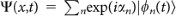, where the Lewis-Riesenfeld phase is given by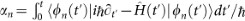and can be understood as the sum of the dynamical phase and the Aharanov-Anandan phase28. For a time-dependent box of width ξ(t) and initial width ξ(0) = ξ0, a dynamical invariant exists31,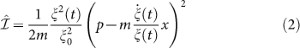with eigenvectors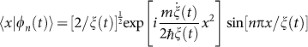and eigenvalues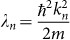, with kn = 0. The Lewis-Riesenfeld phase can be computed to be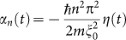with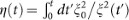. The condition in Eq. (1) for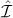to be an invariant requires the box potential to be supplemented with an auxiliary harmonic term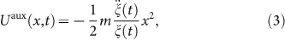whose frequency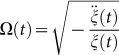is dictated by the ratio of the acceleration of the trajectory ξ(t) and the trajectory itself. Note that ξ(t) > 0, so that for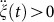, Ω(t) is purely imaginary and the auxiliary term Uaux is a repulsive harmonic potential. If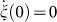,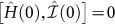so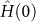and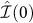have common eigenstates. Further, if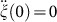holds as well, then Uaux(x, 0) = 0 and an eigenstate Ψn(x, 0) of the box at t = 0 evolves into Ψn(x, t) = exp[n(t)]φn(t), a key observation to engineer a STA as we shall see. We note that the experimental implementation of Uaux(x, t) can be assisted by the same techniques used to create the box potential: the use of a blue-detuned laser32 or direct painting of the required trap15,16,17.

### Shortcuts to adiabaticity: inverting the dynamical scaling law

We next discuss how to implement a non-adiabatic expansion of the box by a factor γ(τ) = ξ(τ)/ξ0 in a given finite-time τ suppressing excitations in the final state. We shall impose the condition Uaux(x, 0) = Uaux(x,τ) = 0. As in the adiabatic case, in a STA the time evolution of an eigenmode of the initial box should reproduce an eigenmode of the final trap. As at t = 0, this can be enforced by imposing the condition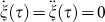. The set of boundary conditions at t = 0, τ excludes the possibility of a linear ramp, as well as the family of trajectories,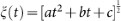, considered so far in the literature25,26,27,28. However, it suffices to determine a polynomial ansatz for the trajectory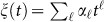, i.e. a scaling factor of the form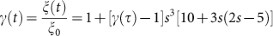, with s = t/τ. This further determines the required time-dependent frequency of the auxiliary harmonic potential Uaux(x, t) according to Eq. (3),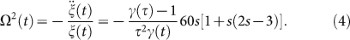The trajectory, displayed in Fig. 1 shows that during an expansion Uaux(x,t) becomes an expulsive potential in an early stage (t < τ/2), providing the speed-up required to achieve the STA in an arbitrary finite time τ (bounds in the presence of perturbations will be discussed below). In a subsequent stage, t > τ/2, Ω2(t) changes sign and Uaux(x, t) becomes a trapping potential, slowing down the expanding mode and reducing it to an eigenstate of the final Hamiltonian at t = τ. Precisely the opposite behavior is exhibited during a fast nonadiabatic compression. Provided that an arbitrary Ω2(t) dependence can be implemented, a STA has no lower bound for τ (notice however that Ω(t) ~ τ–1). By contrast, the adiabatic conditionleads to the requirement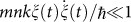.

Note that the energy of the expanding mode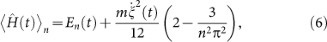has two contributions, the first one being the adiabatic energy En(t) = En(0)ξ(0)2/ξ(t)2 and the second one depending explicitly on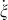, so that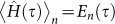given that a STA demands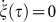. This relation illustrates the fact that a STA is associated with a non-adiabatic evolution, which reproduces the adiabatic result at the end of the process. Moreover, STA work as well for excited states: the time evolution of the n-th eigenstate of the initial trap leads to the n-th eigenstate of the final trap at t = τ. As a result, STA in boxes pave the way for fast population-preserving cooling in the following sense. Given a system described by the canonical ensemble with a density matrix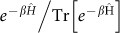, where β = 1/kBT, kB is the Boltzmann constant and T is the temperature, the final temperature reads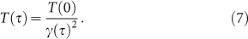These results strictly hold for a box with infinite walls at x [0, ξ(t)]. However, in the all-optical trap reported in18, the end-cap lasers providing the box walls have a Gaussian profile which smooths the potential in a length scale σ, i.e. a box trap of the form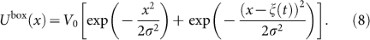A similar smoothing occurs in other physical realizations17. Since this smoothing is expected to be the most significant deviation of laboratory potentials from the ideal infinite box, it is important to consider its effect on the self-similar dynamics required for a STA. This can be quantified by the overlap between the states resulting from the expansion in an idealized and realistic box trap, |Ψn(t)〉 and |Ψ(t)〉 respectively. Clearly the role of σ decreases (increases) during a expansion (contraction) of the box. The numerical solution of the time-dependent Schrödinger equation for this box potential is shown in Fig. 2, where the STA is compared with both the polynomial and linear expansion of the box in the absence of Uaux(x,t). It is seen that the STA is the only successful strategy and that the process is robust even for a substantial smoothing of the potential barriers, where the target states deviate from those of an idealized box.

We have further explored numerically the dynamics under “concatenated STA”, in which the overall expansion is splitted into a sequence of k STA with either constant expansion factor γ or constant box size increment between consecutive steps. The efficiency of the process exhibits a non-monotonic improvement with increasing k, suggesting a natural scenario where STA techniques could be combined with optimal quantum control8,9.

Knowledge of the adiabatic invariants for a single particle in a time-dependent box has provided us with the insight of assisting the dynamics with an auxiliary potential to design STA for expansions and compressions in a finite time, without inducing quantum transients associated with diffraction in time, which are ubiquitous in this type of scenario22. The technique can be directly applied to non-interacting gases and other many-body quantum fluids which can be mapped to non-interacting systems. It further suppresses the Talbot dynamics associated with quantum carpets woven by the density profile typically observed in boxes23 and the question naturally arises as to its applicability to interacting systems24. The presence of interactions, e.g. a two-body potential, hinders the exploitation of the superposition principle in terms of the eigenmodes of the Lewis-Riesenfeld invariant. However, we note that to design a STA it suffices to enforce a self-similar dynamics and ultimately no knowledge of adiabatic invariants is required. As a result, we next consider a broader family of many-body systems, confined in a box, defined by the Hamiltonian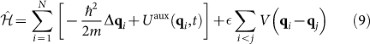where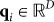, Δqi is the Laplace operator in dimension D, the auxiliary term is now given by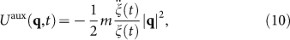and the two-body interaction potential obeys V(λq) = λ–αV(q), e.g. for the Fermi-Huang pseudopotential describing s-wave scattering in ultracold gases, α = D. For a hard-wall box, ri = |q|i [0,ξ(t)]; we shall relax below this approximation and consider realistic potential boxes as those created in all-optical setups. The case D = 1 corresponds to a box with one-wall moving (the symmetric case in which both walls move in opposite directions can be obtained by a Duru transformation33). For D = 2,3 cylindrical and spherical symmetry is assumed respectively. Without loss of generality, we choose the dimensionless time-dependent coupling constant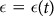to satisfy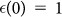. A stationary state Ψ(t) = Ψ(q1, …, qN;t) of N particles and chemical potential μ follows for t > 0 the evolution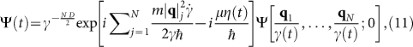with the boundary conditions Ψ(t) = 0 for |q|i = ξ(t) (i = 1,…,N, in addition to Ψ(t) = 0 for |q|i = 0 in D = 1), as long as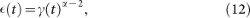which can be implemented exploiting a Feshbach resonance or modulating the transverse confinement in anisotropic systems4,34.

The self-similar dynamics in a STA leads to a scaling of all local correlation functions. In particular the density of a given many-body state follows the law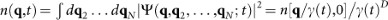. By contrast, non-local correlation functions exhibit a non-trivial dynamics. The one-body reduced density matrix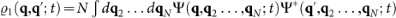of a state obeying Eq. (11), follows the scaling law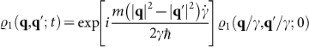, analogous to that observed under harmonic confinement43. The additional phase factor induces a major distortion of the momentum distribution,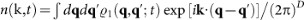. However, a STA ensures that at t = τ the Lewis-Riesenfeld phase factor vanishes, so that the final state exhibits the same correlations of the initial state scaled by a factor γ(τ),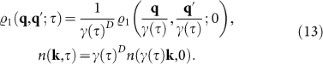### Examples

In the following we shall illustrate different aspects of shortcuts to adiabaticity in some paradigmatic models.

We shall first consider the evolution of correlations in a one-dimensional cloud of ultracold bosons in the limit of hard-core contact interactions, this is, in the Tonks-Girardeau (TG) regime35. This system, as well as its lattice-version, has become a favorite test-bed to study the breakdown of thermalization and adiabaticity36. Its many-body ground state is given by the Bose-Fermi mapping35,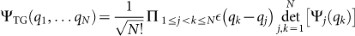, where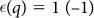if x > 0 (< 0) and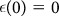. In a STA, the self-similar dynamics is inherited from the single-particle orbitals Ψj(qk, t) whence it follows that no tuning of interactions is required. Its density exhibits a scaling law for all t. The same holds true for the entanglement entropy with respect to a bipartition [0, (t)] (a < 1)37. The self-similar dynamics breaks down for the momentum distribution, which can be computed efficiently38 and we shall focus on its evolution along a STA. Different snapshots are depicted in Fig. 3A and confirm that during an expansion the cloud is accelerated during the interval [0, τ/2] and slowed down during [τ/2, τ]. The reverse sequence, is observed in a fast frictionless compression. The axis are scaled up by the expansion factor γ(t) in such a way that for an adiabatic dynamics, curves at different times would collapse into a single curve. Along a STA, the width and mean of the momentum distribution do not remain constant and change along the process.

A similar distortion of correlations, known as dynamical fermionization, occurs in the dynamics of a cloud suddenly released from an arbitrary trap39. Under ballistic dynamics the asymptotic momentum distribution in a 1D expansion evolves to that of the dual system, a spin polarized Fermi gas. In particular for a cloud released from a box the exact time evolution is not self-similar40 but dynamical fermionization is observed41,42. However, under a self-similar scaling law, the asymptotic n(k) maps to the density profile of the initial state43 and no dynamical fermionization occurs. This is the case of relevance to STA, where the dynamical scaling law in Eq. (11) holds. (We note that the case of the initial harmonic confinement is singular in that the free expansion is self-similar and that the the single-particle eigenstates can be written in terms of Hermite polynomials, which are eigenfunctions of the continuous Fourier transform. As a result the asymptotic momentum distribution can be related to both the initial density profile and the momentum distrubtion of non-interacting fermions. See  for a discussion of STA in harmonic traps.) Moreover, this distortion of correlations is not restricted to expansion processes. Along a STA, this is shown in Fig. 3 for both expansions (A) and compressions (B). This is a spurious effect for the purpose of STA, which is to reproduce the adiabatic result in a finite short time. Indeed, the distortion induced during the first half of the STA associated with the accelerated expansion or compression, is compensated in the second half of the dynamics, in such a way that the correlations of the initial state are reconstructed at t = τ and scaled by a factor γ(τ).

We next turn our attention to the design of STA for a BEC in time-dependent box trap, where different strategies can be adopted depending on the dimensionality and the regime of interactions. The time-dependent Gross-Pitaevski equation (TDGPE) governs the evolution of the normalized condensate wavefunction Φ(q, t),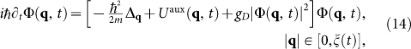for which adiabaticity conditions have been reported29. The ansatz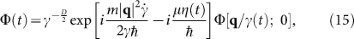satisfies the TDGPE provided that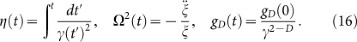These relations constitute the box analogue of the well-known Castin-Dum-Kagan-Surkov-Shlyapnikov relations in harmonic traps44,45. The two-dimensional case is special since the scaling law holds when g2D(t) is kept constant.

Figure 4 is a set of numerical solutions of the time-dependent Gross-Pitaeveskii equation that illustrate the robustness of the STA for realistic BEC experiments. We consider a box trap with Gaussian barriers and in all numerical simulations interactions are kept constant, i.e. gD(t) = gD(0), deviating from the ideal prescription in Eq. (16). The top row illustrates the dynamics for a quasi-1D BEC. The (one-body) fidelity between the resulting state Φ(τ) and the ground state of the final box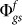is. For smaller values of γ, the fidelity is even higher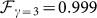as expected, given that implementation of the exact STA requires a smaller tuning of g1. The bottom row shows the dynamics of a quasi-2D cloud, which requires no interaction tuning in a STA, but is more sensitive to the smoothness of the box boundaries,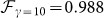.